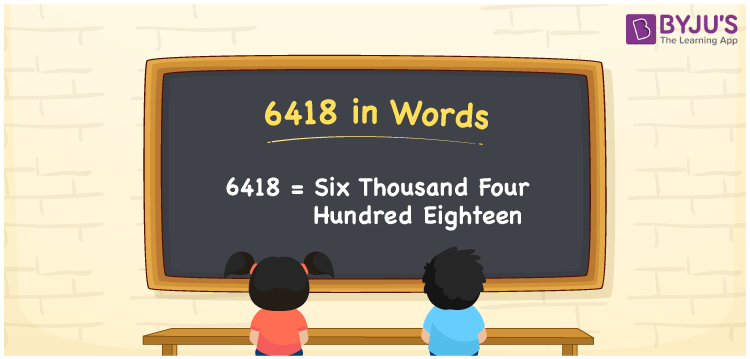# 6418 in Words

6418 in words is “Six Thousand Four Hundred Eighteen”. For example, if you spent Rs. 6418 on purchasing shirts, you can write it as “I spent Rs. Six thousand four hundred eighteen on purchasing shirts”. Also, 6418 is a cardinal number. In this article, let us discuss how to write the spelling of the number 6418 in words using the place value system.

 6418 in Words: Six Thousand Four Hundred Eighteen. Six Thousand Four Hundred Eighteen in Numerical Form: 6418.

## 6418 in English Words## How to Write 6418 in Words?

Following is the place value table for the number 6418.

 Thousands Hundreds Tens Ones 6 4 1 8

The expanded form of 6418 is as follows:

= 6 × Thousand + 4 × Hundred + 1 × Ten + 8 × One

= 6 × 1000 + 4 × 100 + 1 × 10 + 8 × 1

= 6000 + 400 + 10 + 8

= 6418

= Six thousand four hundred eighteen

Hence, 6418 in words is six thousand four hundred eighteen.

The number 6418 exists between 6417 and 6419.

6418 in words – Six thousand four hundred eighteen

Is 6418 an odd number? – No

Is 6418 an even number? – Yes

Is 6418 a perfect square number? – No

Is 6418 a perfect cube number? – No

Is 6418 a prime number? – No

Is 6418 a composite number? – Yes

## Frequently Asked Questions on 6418 in Words

Q1

### How to spell 6418?

6418 in words is six thousand four hundred eighteen.

Q2

### Simplify 6400 + 18, and express it in words.

Simplifying 6400 + 18, we get 6418. Hence, 6418 in words is six thousand four hundred eighteen.

Q3

### Convert six thousand four hundred eighteen into numbers.

Six thousand four hundred eighteen in numbers is 6418.